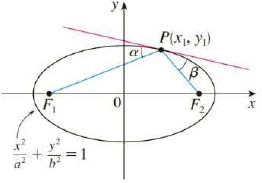Chapter 10.5, Problem 65E

Chapter
Section
Textbook Problem

# Let P(x1, y1) be a point on the ellipse x2/a2 + y2/b2 = 1 with foci F1 and F2 and let α and β be the angles between the lines PF1, PF2 and the ellipse as shown in the figure. Prove that α = β. This explains how whispering galleries and lithotripsy work. Sound coming from one focus is reflected and passes through the other focus. [Hint: Use the formula in Problem 17 on page 201 to show that tan α = tan β.]To determine

To prove: The angles between the lines are equal. That is, α=β

Explanation

Given:

The equation of ellipse is x2a2+y2b2=1 (1)

Calculation:

Differentiate the equation (1) with respect to x

x2a2+y2b2=12xa2+2y.y'b2=12y.y'b2=2xa2y'=2xb22a2yy'=xb2ya2

Therefore, the slope of tangent at point P (x1,y1) is m3=x1b2y1a2

Calculate the slopes at F1P

Here, F1 is at (c,0) and P is at (x1,y1)

F1P(m1)=y2y1x2x1=0y1cx1=y1(c+x1)=y1c+x1

Calculate the slopes at F1P

Here, F1 is at (0,c) and P is at (x1,y1)

F2P(m2)=y2y1x2x1=y10x1c=y1x1c

Consider the ellipse equation.

x12a2+y12b2=1x12b2+y12a2a2b2=1x12b2+y12a2=a2b2

Calculate the angle tanα

tanα=m1m31+m3m1=y1x1+c(x1b2y1a2)1+[(y1x1+c)(x1b2y1a2)]=y1x1+c+x1b2y1a21[(y1x1+c)(x1b2y1a2)]

tanα=y1(y1a2)+x1b2(x1+c)(x1+c)y1a21y1x1b2(x1+c)y1a2=y1(y1a2)+x1b2(x1+c)(x1+c)y1a2(y1a2(x1+c))y1x1b2(x1+c)y1a2=y1(y1a2)+x1b2(x1+c)(x1+c)y1a2×(x1+c)y1a2(y1a2(x1+c))y1x1b2

tanα=y1(y1a2)+x1b2(x1+c)(y1a2(x1+c))y1x1b2=a2y12+b2x12+x1b2cx1y1a2+y1a2cx1y1b2=a2y12+b2x12+x1b2cx1y1(a2b2)+y1a2c

Substitute c2 for a2b2 and a2b2 for x12b2+y12a2 in the above equation

### Still sussing out bartleby?

Check out a sample textbook solution.

See a sample solution

#### The Solution to Your Study Problems

Bartleby provides explanations to thousands of textbook problems written by our experts, many with advanced degrees!

Get Started

#### In Exercises 13-20, sketch a set of coordinate axes and plot each point. 16. (3, 4)

Applied Calculus for the Managerial, Life, and Social Sciences: A Brief Approach

#### Find the derivatives of the functions in Problems 1-34. 13.

Mathematical Applications for the Management, Life, and Social Sciences

#### For , f′(x) =

Study Guide for Stewart's Multivariable Calculus, 8th

#### Evaluate each expression. k=1106

College Algebra (MindTap Course List)